Courses

# RD Sharma Solutions (Part - 1) - Ex-21.1, Mensuration - II Area of Circle, Class 7, Math Class 7 Notes | EduRev

## Class 7: RD Sharma Solutions (Part - 1) - Ex-21.1, Mensuration - II Area of Circle, Class 7, Math Class 7 Notes | EduRev

The document RD Sharma Solutions (Part - 1) - Ex-21.1, Mensuration - II Area of Circle, Class 7, Math Class 7 Notes | EduRev is a part of the Class 7 Course RD Sharma Solutions for Class 7 Mathematics.
All you need of Class 7 at this link: Class 7

#### Question 1:

Find the circumference of a circle whose radius is
(i) 14 cm
(ii) 10 m
(iii) 4 km

(i) The circumference C of a circle of radius 'r' is given by:
C = 2πr
Here, r = 14 cm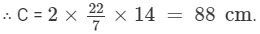(ii) The circumference C of a circle of radius 'r' is given by:

C = 2πr
Here, r = 10 m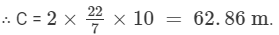(iii) The circumference C of a circle of radius 'r' is given by:
C = 2πr
Here, r = 4 km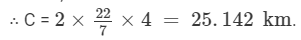#### Question 2:

Find the circumference of a circle whose diameter is
(i) 7 cm
(ii) 4.2 cm
(iii) 11.2 km

(i) We have:
Diameter = 7 cm
Let C be the circumference of a circle. Then,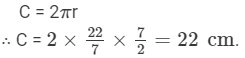(ii)   We have:
Diameter = 4.2 cm
∴ Radius = 4.2/2 cm= 2.1 cm
Let C be the circumference of a circle. Then,
C = 2ππr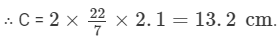(iii) We have:
Diameter = 11.2 km
∴ Radius = 11.2/2 km= 5.6 km
Let C be the circumference of a circle. Then,
C = 2πr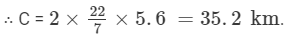#### Question 3:

Find the radius of a circle whose circumference is
(i) 52.8 cm
(ii) 42 cm
(iii) 6.6 km

(i) We know that the circumference C of a circle of  radius 'r' is given by:
C = 2πr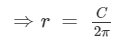Here, C = 52.8 cm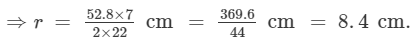(ii) We know that the circumference C of a circle of radius 'r' is given by:
C = 2πrHere, C = 42 cm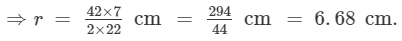(iii) We know that the circumference C of a circle of radius 'r' is given by:
C = 2πrHere, C = 6.6 km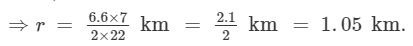#### Question 4:

Find the diameter of a circle whose circumference is
(i) 12.56 cm
(ii) 88 m
(iii) 11.0 km

(i) We know that the circumference C of a circle of  diameter 'd' is given by:
C = πd

⇒d = C/π
Here, C = 12.56 cm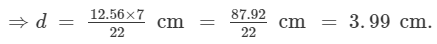(ii) We know that the circumference C of a circle of  diameter 'd' is given by:
C = πd

⇒d = C/π
Here, C = 88 m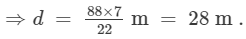(iii) We know that the circumference C of a circle of diameter 'd' is given by:
C = πd
⇒d = C/π
Here, C = 11 km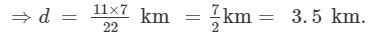#### Question 5:

The ratio of the radii of two circles is 3 : 2. What is the ratio of their circumferences?

We have, the ratio of the radii = 3:2
So, let the radii of the two circles be 3r and 2r, respectively.
Let C1 and C2 be the circumferences of the two circles of radii 3r and 2r, respectively. Then,
C1= 2πx 3r = 6πr,  and C2 = 2πx2r = 4πr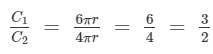or
C1:C2 = 3:2

#### Question 6:

A wire in the form of a rectangle 18.7 cm long and 14.3 cm wide is reshaped and bent into the form of a circle. Find the radius of the circle so formed.

Length of the wire = Perimeter of the rectangle
= 2 ( l +) = 2 x (18.7 + 14.3) = 66 cm
Let the wire be bent in the form of a circle of radius r cm. Then,
circumference = 66 cm

⇒2πr = 66 cm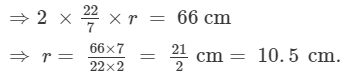#### Question 7:

A piece of wire is bent in the shape of an equilateral triangle of each side 6.6 cm. it is re-bent to form a circular ring. What is the diameter of the ring?

We have:
Length of the wire = The perimeter of  the equilateral triangle

=  3 x side = 3 x 6.6 =   19.8 cm.
Let the wire be bent to form a circular ring of radius r cm.Then,
Circumference =  19.8 cm
⇒2πr = 19.8 cm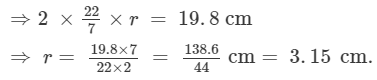So, the diameter of the ring = 2 x 3.15 = 6.30 cm.

#### Question 8:

The diameter of a wheel of a car is 63 cm. Find the distance travelled by the car during the period, the wheel makes 1000 revolutions.

It may be noted that in one revolution, the cycle covers a distance equal to the circumference of the wheel.
Now, the diameter of the wheel = 63 cm

∴ Circumference of the wheel = πd   =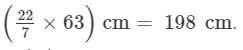Thus, the cycle covers 198 cm in one revolution.
∴ The distance covered by the cycle in 1000 revolutions = (198 x 1000 ) cm = 198000 cm = 1980 m.

#### Question 9:

The diameter of a wheel of a car is 98 cm. How many revolutions will it make to travel 6160 metres.

We have:
Diameter of the wheel of the car = 98 cm
∴ Circumference of the wheel of the car = πd =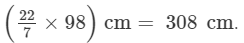Note that, in one revolution of the wheel, the car travels a distance equal to the circumference of the wheel.

∴ The distance travelled by the car in one revolution of the wheel = 308 cm.
Total distance travelled by the car = 6160 m = 616000 cm.
∴ Number of revolutions =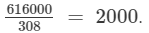#### Question 10:

The moon is about 384400 km from the earth and its path around the earth is nearly circular. Find the circumference of the path described by the moon in lunar month.

We have:
The radius of the path described by the moon around the earth = 384400 km
∴ The circumference of the path described by the moon C =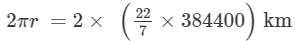= 2416228.57 km

The document RD Sharma Solutions (Part - 1) - Ex-21.1, Mensuration - II Area of Circle, Class 7, Math Class 7 Notes | EduRev is a part of the Class 7 Course RD Sharma Solutions for Class 7 Mathematics.
All you need of Class 7 at this link: Class 7Use Code STAYHOME200 and get INR 200 additional OFF Use Coupon Code
All Tests, Videos & Notes of Class 7: Class 7

### Top Courses for Class 7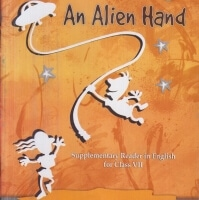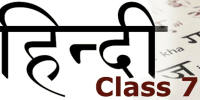## RD Sharma Solutions for Class 7 Mathematics

97 docs

### Top Courses for Class 7Track your progress, build streaks, highlight & save important lessons and more!

,

,

,

,

,

,

,

,

,

,

,

,

,

,

,

,

,

,

,

,

,

,

,

,

,

,

,

,

,

,

;